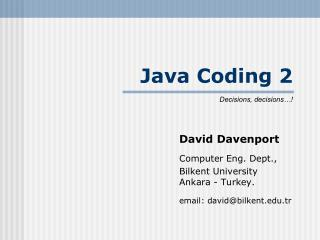# Java Coding 2 - PowerPoint PPT PresentationDownload PresentationJava Coding 2

Java Coding 2
Download Presentation## Java Coding 2

- - - - - - - - - - - - - - - - - - - - - - - - - - - E N D - - - - - - - - - - - - - - - - - - - - - - - - - - -
##### Presentation Transcript

1. Java Coding 2 Decisions, decisions…! David Davenport Computer Eng. Dept., Bilkent UniversityAnkara - Turkey. email: david@bilkent.edu.tr

2. IMPORTANT… • Students… This presentation is designed to be used in class as part of a guided discovery sequence. It is not self-explanatory! Please use it only for revision purposes after having taken the class. Simply flicking through the slides will teach you nothing. You must be actively thinking, doing and questioning to learn! • Instructors… You are free to use this presentation in your classes and to make any modifications to it that you wish. All I ask is an email saying where and when it is/was used. I would also appreciate any suggestions you may have for improving it. thank you,David.

3. Decision • The Java if statement if (condition) statementT; if (condition) statementT; else statementF; • where • statement is any Java statement • condition is a boolean expression

4. Conditions • Any expression with Boolean result • boolean variable • canVote taxable found • Method with boolean result • exists( filename) isSent( myEmail) • Operand relationalOperator Operand(where relationalOperator is: >, <, >=, <=, ==, != ) • age >= 18 speed != 0 year % 4 == 0 • Boolean expressions combined with logicalOperator(where logicalOperator is: &&, ||, ! ) • height > 2 && weight <= 80 x < 5 || x > 10 • ! exists( filename) aChar >= ‘0’ && aChar <= ‘9’

5. Examples (1) • Print message when x is positive • if ( x > 0 ) System.out.println( “The value of x is positive”); • Print warning if oil pressure is below a specified limit • if ( oilPressure < MINIMIUM_OIL_PRESSURE ) System.out.println( “Warning – low !”); • Report whether x is negative or not • if ( x < 0 )System.out.println( “Negative”);elseSystem.out.println( “Positive or zero”); • Rewrite with alternative condition • Check user’s password • if ( !actualPassword.equals( enteredPassword) ) System.out.println( “Sorry, incorrect Password”);else // do secure things!

6. Examples (2) • Compute z as absolute value of x-y • if ( x – y < 0 ) z = y – x;else z = x – y; • if ( x > y ) z = x - y;else z = y - x; • z = x – y;if ( z < 0 ) z = -z; Can also use z = Math.abs(x-y);

7. Examples (3) • Given three values stored in variables first, second, third, store the minimum in a variable called min • if ( first < second ) min = first;else min = second;if ( third < min ) min = third; • Generalise…?

8. { statement; statement; statement; }  statement; Examples (4) • Avoid divide-by-zero errors • if ( y = 0 ) System.out.println( “Error: can’t divide by zero”);else z = x / y; System.out.println( “The result is “ + z ); • Use braces (curly brackets) to form compound statement

9. Examples (5) • Choosing between three alternatives: • if ( x < 0 ) System.out.println( “Negative”);else { if ( x == 0 ) System.out.println( “Zero”); else System.out.println( “Positive”);} • if ( x >= 0 ) { if ( x == 0 ) System.out.println( “Zero”); else System.out.println( “Positive”);}else System.out.println( “Negative”);

10. Examples (6) • A neater way of writing mutually exclusive alternatives (nested if): • if ( x < 0 ) System.out.println( “Negative”);else if ( x == 0 ) System.out.println( “Zero”);else if ( x < 5) System.out.println( “1 – 4 inclusive”);else System.out.println( “>= 5”); // & x >= 0 // & x >= 0 & x != 0 // & x >= 0 & x != 0 & x >= 5

11. Distinguish… if (cond) print “A”else if (cond) print “B”else if (cond) print “C”else print “D” if (cond) print “A”if (cond) print “B”if (cond) print “C”if (cond) print “D”